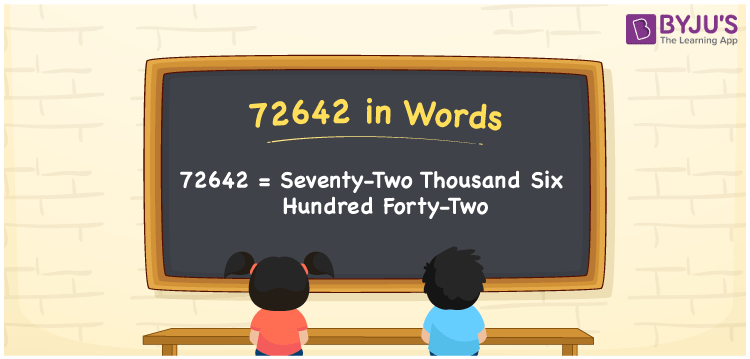# 72642 in Words

72642 in words is written as “seventy-two thousand six hundred forty-two”. For example, the cheque of 72642 is written as Rs. Seventy-two thousand six hundred forty-two only. In Mathematics, the number 72642 is a cardinal number as it represents the quantity of some objects.

 72642 in Words: Seventy-two Thousand Six Hundred Forty-two. Seventy-two Thousand Six Hundred Forty-two in Numerical Form: 72642.

## 72642 in English Words## How to Write 72642 in Words?

Learn the place values of each digit of the number 72642 and write the number in words.

 Ten-thousands Thousands Hundreds Tens Ones 7 2 6 4 2

The expanded form of 72642 is as follows:

= 7 × Ten thousand + 2 × Thousand + 6 × Hundred + 4 × Ten + 2 × One

= 7× 10000 + 2 × 1000 + 6 × 100 + 4 × 10 + 2 × 1

= 70000 + 2000 + 600 + 40 + 2

= 72642

= Seventy-two thousand six hundred forty-two

Hence, 72642 in words is seventy-two thousand six hundred forty-two.

72642 in words – Seventy-two thousand six hundred forty-two

Is 72642 an odd number? – No

Is 72642 an even number? – Yes

Is 72642 a perfect square number? – No

Is 72642 a perfect cube number? – No

Is 72642 a prime number? – No

Is 72642 a composite number? – Yes

## Frequently Asked Questions on 72642 in Words

Q1

### How to write 72642 in English words?

72642 in words is seventy-two thousand six hundred forty-two.

Q2

### Simplify 72000 + 642, and express it in words.

Simplifying 72000 + 642, we get 72642. Hence, 72642 in words is seventy-two thousand six hundred forty-two.

Q3

### Is 72642 an even number?

Yes, 72642 is an even number.